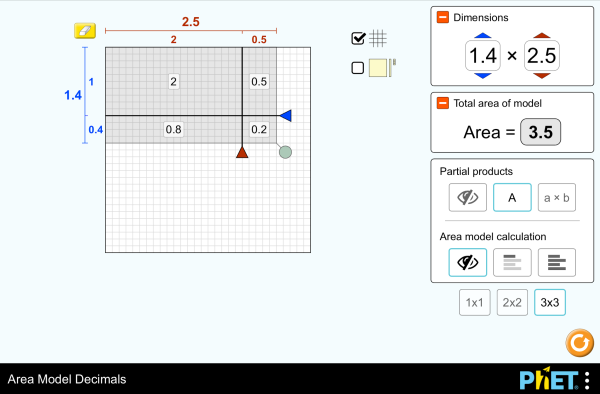# Area Model Decimals_面積模型與小數下載嵌入 關閉 嵌入模擬教學執行複本 利用 HTML 來嵌入模擬教學執行複本。您可以在 HTML 中改變已嵌入模擬教學的寬度及高度。 嵌入啓動模擬教學之圖樣

### 標題

• Multiplication
• Decimals
• Area Model
• Factors
• Partial Products

### 學習目標

• Recognize that area represents the product of two numbers and is additive.
• Develop and justify a strategy that uses the area model to simplify a decimal multiplication problem.
• Devise a strategy to determine the product of two rational numbers (or an integer and a rational number) by representing the product as an area or the sum of areas.
• Use an area model to understand why multiplying a given number by a decimal less than 1 results in a product smaller than the given number.
• Interpret the product of two rational numbers using base-ten tiles

### 標準對齊

#### 共用核心 - 數學

5.NF.B.4
Apply and extend previous understandings of multiplication to multiply a fraction or whole number by a fraction.
5.NF.B.4a
Interpret the product (a/b) × q as a parts of a partition of q into b equal parts; equivalently, as the result of a sequence of operations a × q ÷ b. For example, use a visual fraction model to show (2/3) × 4 = 8/3, and create a story context for this equation. Do the same with (2/3) × (4/5) = 8/15. (In general, (a/b) × (c/d) = ac/bd.)
5.NF.B.4b
Find the area of a rectangle with fractional side lengths by tiling it with unit squares of the appropriate unit fraction side lengths, and show that the area is the same as would be found by multiplying the side lengths. Multiply fractional side lengths to find areas of rectangles, and represent fraction products as rectangular areas.
5.NF.B.5
Interpret multiplication as scaling (resizing), by:
5.NF.B.5a
Comparing the size of a product to the size of one factor on the basis of the size of the other factor, without performing the indicated multiplication.
5.NF.B.5b
Explaining why multiplying a given number by a fraction greater than 1 results in a product greater than the given number (recognizing multiplication by whole numbers greater than 1 as a familiar case); explaining why multiplying a given number by a fraction less than 1 results in a product smaller than the given number; and relating the principle of fraction equivalence a/b = (n × a)/(n × b) to the effect of multiplying a/b by 1.

### 老師提供的活動

Games Remote Lesson ideasTrish Loeblein K-5

Remote

Area Model Decimals - Grade 6Maura Dudley 國中 指引 數學
PRIMARIA: Alineación con programas de la SEP México (2011 y 2017)Diana López 國中
K-5

Middle School Math Sim AlignmentAmanda McGarry 國中 其它 數學

Serbian 全部 СрпскиМатематика преко површина-Децимални бројеви
Sinhalese 全部 Sinhaleseවර්ගඑල ආකෘති දශම
Tamil 全部 Tamilமாதிரிகளின் தசமப் பரப்பளவு

HTML5 sims can run on iPads and Chromebooks, as well as PC, Mac, and Linux systems.

iOS 12+ Safari

Android:
Not officially supported. If you are using the HTML5 sims on Android, we recommend using the latest version of Google Chrome.

Chromebook:
The HTML5 and Flash PhET sims are supported on all Chromebooks.
Chromebook compatible sims

Windows Systems:

Macintosh Systems:

Linux Systems:

• Jonathan Olson (developer)
• Karina Hensberry
• Susan Miller
• Ariel Paul
• Kathy Perkins
• almond-0.2.9.js
• base64-js-1.2.0.js
• FileSaver-b8054a2.js
• font-awesome-4.5.0
• game-up-camera-1.0.0.js
• he-1.1.1.js
• himalaya-0.2.7.js
• jama-1.0.2
• jquery-2.1.0.js
• lodash-4.17.4.js
• pegjs-0.7.0.js
• seedrandom-2.4.2.js
• text-2.0.12.js
• TextEncoderLite-3c9f6f0.js